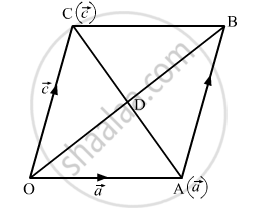Share
Notifications

View all notifications
Books Shortlist
Your shortlist is empty

# Prove that the Diagonals of a Rhombus Are Perpendicular Bisectors of Each Other. - CBSE (Arts) Class 12 - Mathematics

Login
Create free account

Forgot password?

#### Question

Prove that the diagonals of a rhombus are perpendicular bisectors of each other.

#### SolutionLet OABC be a rhombus, whose diagonals OB and AC intersect at D. Suppose O is the origin.

Let the position vector of A and C be  $\vec{a}$ and $\vec{c}$ respectively.

Then, $\vec{OA} = \vec{a}$

In ∆OAB,

$\vec{OB} = \vec{OA} + \vec{AB} = \vec{OA} + \vec{OC} = \vec{a} + \vec{c}$..........$\left( \vec{AB} = \vec{OC} \right)$

Position vector of mid-point of $\vec{OB} = \frac{1}{2}\left( \vec{a} + \vec{c} \right)$

Position vector of mid-point of$\vec{OB} = \frac{1}{2}\left( \vec{a} + \vec{c} \right)$................(Mid-point formula)

So, the mid-points of OB and AC coincide. Thus, the diagonals OB and AC bisect each other.

Now,

$\vec{OB} . \vec{AC} = \left( \vec{a} + \vec{c} \right) . \left( \vec{c} - \vec{a} \right)$
$= \left( \vec{c} + \vec{a} \right) . \left( \vec{c} - \vec{a} \right)$
$= \left| \vec{c} \right|^2 - \left| \vec{a} \right|^2$
$= \left| \vec{OC} \right|^2 - \left| \vec{OA} \right|^2$
$= 0 \left( \left| \vec{OC} \right| = \left| \vec{OA} \right| \right)$
$\Rightarrow \vec{OB} \perp \vec{AC}$

Hence, the diagonals OB and AC are perpendicular to each other.

Thus, the diagonals of a rhombus are perpendicular bisectors of each other.

Is there an error in this question or solution?

#### Video TutorialsVIEW ALL 

Solution Prove that the Diagonals of a Rhombus Are Perpendicular Bisectors of Each Other. Concept: Section formula.
S